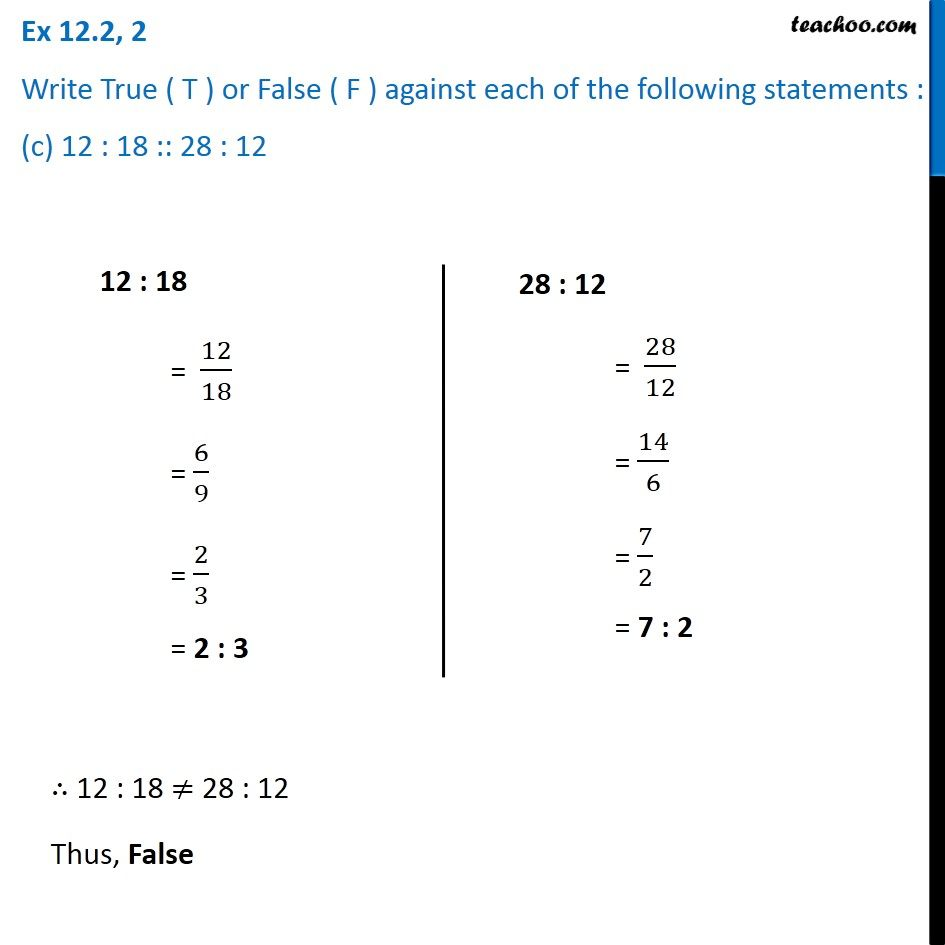Ex 12.2

Chapter 12 Class 6 Ratio and Proportion
Serial order wiseLearn in your speed, with individual attention - Teachoo Maths 1-on-1 Class

### Transcript

Ex 12.2, 2 Write True ( T ) or False ( F ) against each of the following statements : (c) 12 : 18 :: 28 : 1212 : 18 = 12/18 = 6/9 = 2/3 = 2 : 3 28 : 12 = 28/12 = 14/6 = 7/2 = 7 : 2 ∴ 12 : 18 ≠ 28 : 12 Thus, False